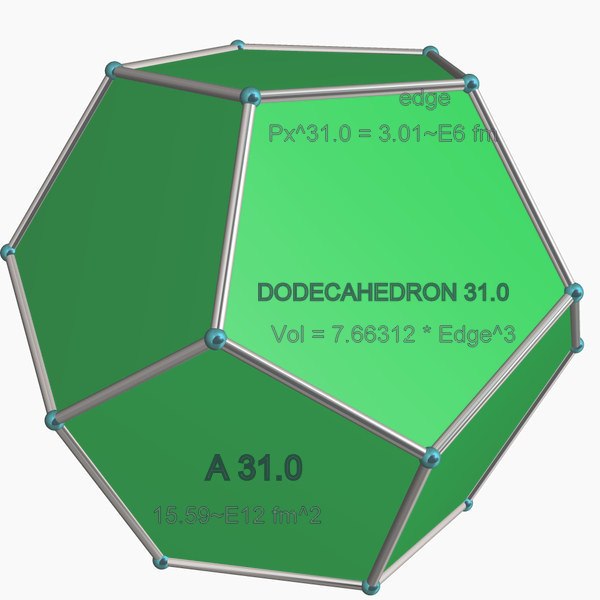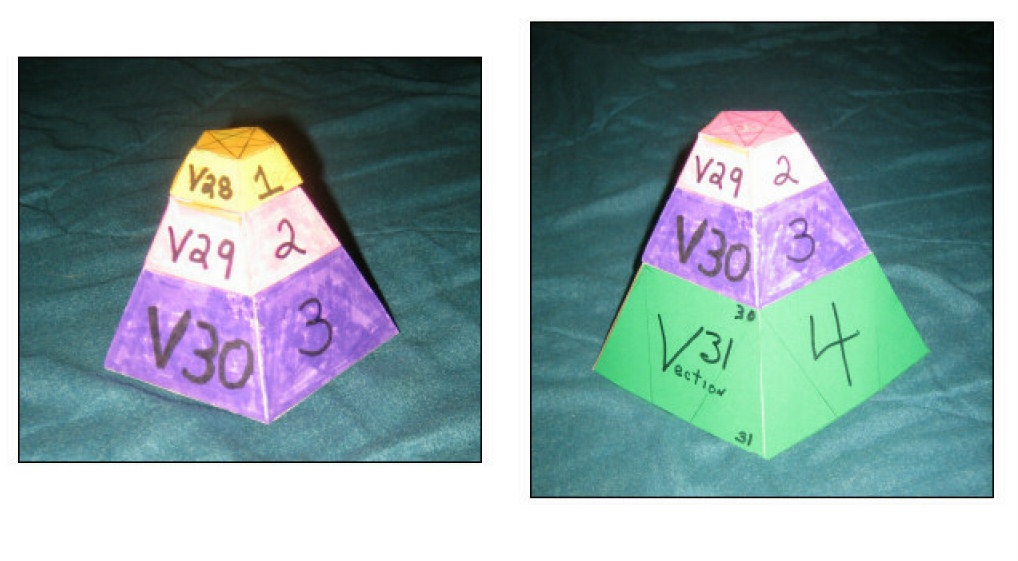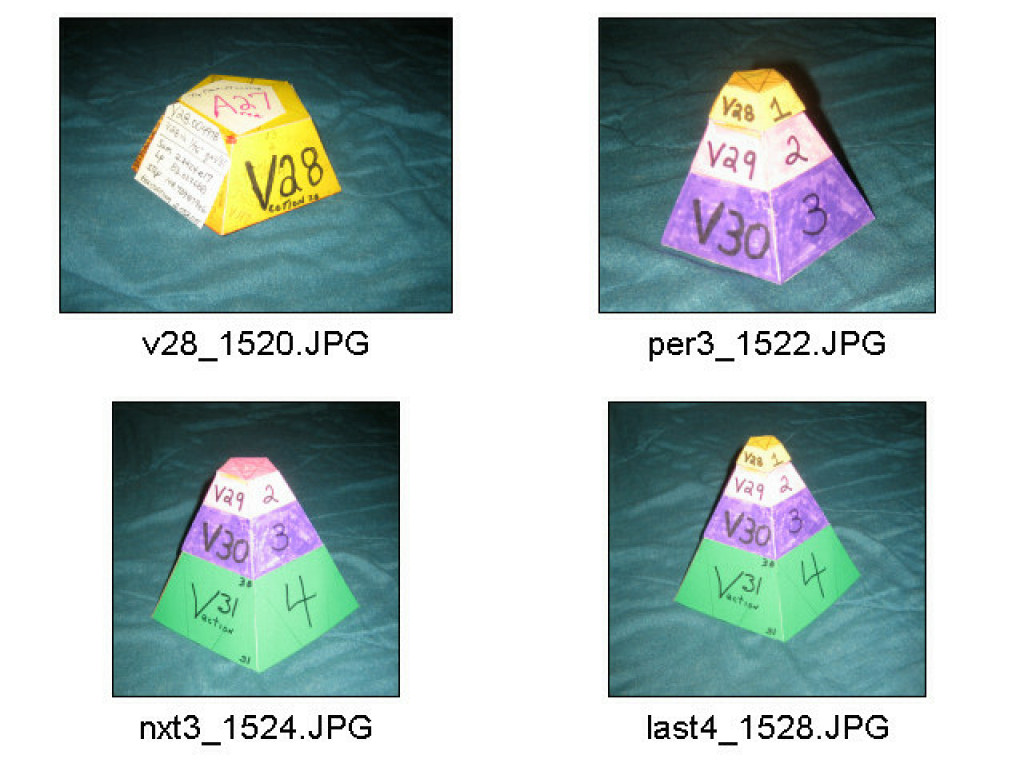Preliminary Construction Proof of Prime Numbers having a cubic nature!

-----IN PROGRESS ------------------Mar 2015---

### "Construction" Proof Relevance

Aside from the elementary summing , there are no equations involved except a simple curve fit that extrapolates to higher estimates in order to show that the running sum of the Prime Numbers using Lp, the Golden Ratio logs, continues indefinitely, in an obviously orderly manner.

The simplicity of the proof and its graphs should allow for an opinion of correctness at least; significance can be dealt with later out of fairness to the Data presented which can be re-generated on any computer, by simply summing the PN's upward and recording the STEP,PN,SUM trio at unit Lp steps.

### Geometry: Graphs and Methods

1. Chose a volume amount; assign it to that of a Dodecahedron ;

2. Compute the Dodecahedron's EDGE value from ( Volume / 7.66312 )^-3 ; its Height or Width was its Edge times the Square root of 5.

3. The Lp of the EDGE value is the Top Plane value and the Area index for the face.

4. A Tone volume is geometrically a 12th of the dodecahedron's volume; it was made of layers of Vections corresponding to the step pattern labeled in the table by Top Plane / EDGE values.

---------- Since the Volume of a Dodecahedron equals "12 * PNsum" , thus making its EDGE equal to (12 / 7.66312 * PNsum )^-3 !

5. For example, at table step 8749 of PN:90371 with Sum:3.741730820e+08 and its Lp:41.021912 & TOP Plane:A14.004849.

Multiplying the sum by "12 / 7.66312" gives a volume of 58.593273e6 with its cube root of 836.79~
which translates to an 13.984~ Top Plane value which is more accurate than the 14.04 derived from just using the last three sums.------------------- Vections ------------------